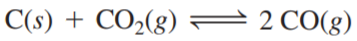×
Get Full Access to Chemistry: A Molecular Approach - 3 Edition - Chapter 14 - Problem 69e
Get Full Access to Chemistry: A Molecular Approach - 3 Edition - Chapter 14 - Problem 69e

×

# This reaction is endothermic. Predict the effect (shiftISBN: 9780321809247 1

## Solution for problem 69E Chapter 14

Chemistry: A Molecular Approach | 3rd Edition

• Textbook Solutions
• 2901 Step-by-step solutions solved by professors and subject experts
• Get 24/7 help from StudySoup virtual teaching assistantsChemistry: A Molecular Approach | 3rd Edition

4 5 1 418 Reviews
13
3
Problem 69E

This reaction is endothermic.Predict the effect (shift right, shift left, or no effect) of increasing and decreasing the reaction temperature. How does the value of the equilibrium constant depend on temperature?

Step-by-Step Solution:

Solution 69EStep 1The given reaction is endothermic.Endothermic reactions are those which take up the energy from the surroundings.In such a case heat is considered as one of the reactant. Now the chemical reaction can be writtenasIn the above reaction reactants and products are present in different phases.

Step 2 of 3

Step 3 of 3

##### ISBN: 9780321809247

Since the solution to 69E from 14 chapter was answered, more than 377 students have viewed the full step-by-step answer. This textbook survival guide was created for the textbook: Chemistry: A Molecular Approach, edition: 3. The full step-by-step solution to problem: 69E from chapter: 14 was answered by , our top Chemistry solution expert on 02/22/17, 04:35PM. Chemistry: A Molecular Approach was written by and is associated to the ISBN: 9780321809247. This full solution covers the following key subjects: temperature, shift, reaction, effect, increasing. This expansive textbook survival guide covers 82 chapters, and 9454 solutions. The answer to “?This reaction is endothermic. Predict the effect (shift right, shift left, or no effect) of increasing and decreasing the reaction temperature. How does the value of the equilibrium constant depend on temperature?” is broken down into a number of easy to follow steps, and 32 words.

Unlock Textbook Solution